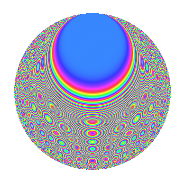# Properties

 Label 1575.2.cmLevel 1575 Weight 2 Character orbit cm Rep. character $$\chi_{1575}(107,\cdot)$$ Character field $$\Q(\zeta_{12})$$ Dimension 192 Sturm bound 480

# Related objects

## Defining parameters

 Level: $$N$$ = $$1575 = 3^{2} \cdot 5^{2} \cdot 7$$ Weight: $$k$$ = $$2$$ Character orbit: $$[\chi]$$ = 1575.cm (of order $$12$$ and degree $$4$$) Character conductor: $$\operatorname{cond}(\chi)$$ = $$105$$ Character field: $$\Q(\zeta_{12})$$ Sturm bound: $$480$$

## Dimensions

The following table gives the dimensions of various subspaces of $$M_{2}(1575, [\chi])$$.

Total New Old
Modular forms 1056 192 864
Cusp forms 864 192 672
Eisenstein series 192 0 192

## Trace form

 $$192q - 8q^{7} + O(q^{10})$$ $$192q - 8q^{7} + 96q^{16} + 48q^{22} - 88q^{28} + 16q^{31} + 16q^{37} + 16q^{43} + 80q^{52} + 88q^{58} - 56q^{61} + 32q^{67} + 88q^{73} + 320q^{76} + 56q^{82} - 120q^{88} - 104q^{91} - 208q^{97} + O(q^{100})$$

## Decomposition of $$S_{2}^{\mathrm{new}}(1575, [\chi])$$ into newform subspaces

The newforms in this space have not yet been added to the LMFDB.

## Decomposition of $$S_{2}^{\mathrm{old}}(1575, [\chi])$$ into lower level spaces

$$S_{2}^{\mathrm{old}}(1575, [\chi]) \cong$$ $$S_{2}^{\mathrm{new}}(105, [\chi])$$$$^{\oplus 4}$$$$\oplus$$$$S_{2}^{\mathrm{new}}(315, [\chi])$$$$^{\oplus 2}$$$$\oplus$$$$S_{2}^{\mathrm{new}}(525, [\chi])$$$$^{\oplus 2}$$

## Hecke Characteristic Polynomials

There are no characteristic polynomials of Hecke operators in the database